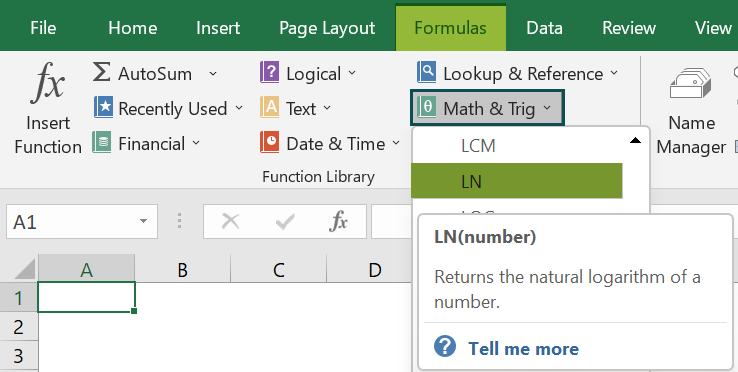# LN Excel FunctionArticle byExcelMojo Team## What Is LN Function In Excel?

The LN Function in Excel calculates the natural logarithm of a given number. The function is categorized under the Math & Trig function from the Function Library.

The LN Function denotes the logarithm statement, and as per the syntax, we put the number of logarithm values we need to find; we can choose any exponential or raise to power value in the syntax. For example, consider the below table with a value in cell A1. We need to use the below steps to obtain the result with LN Excel Function.

The steps used to find the log value in Excel are as follows. To begin with, select the cell to display the result. In this example, we have selected cell A1. Next, enter the LN formula in cell A1. So, the complete formula is =LN(2). Then, press Enter key. We can clearly see that the function has returned the log value in cell A1.

Similarly, we can use LN function to fetch the log value.

###### Key Takeaways
• The LN Excel Function is used to find the log values.
• The LN Excel formula is =LN(number) where, number denotes the number we assign to get the natural logarithm number.
• The natural logarithm is related to the EXP function. In EXP, the value of a base number is raised to an exponential number; in the logarithm, so, we need to know the exponential number to get the desired number.
• If the parameter is a negative number, it returns an error with #NUM! thus, indicating an error with the number.

### LN() Excel Formula

The syntax of the LN Excel formula is

where, number is the mandatory argument and it denotes the number we assign to get the natural logarithm number.

### How To Use LN Excel Function?

We can insert LN Excel function using the below two methods.

#### Method #1 – Access From The Excel Ribbon

• To begin with, choose the empty cell which will contain the result.
• Next, go to the Formulas tab.
• Then, select the Math & Trig option from the menu.
• Similarly, select LN from the drop-down menu.
• A window called Function Arguments appears.
• As the number of arguments, enter the value in the number.
• Select OK.

#### Method #2 – Enter The Worksheet Manually

• Select an empty cell for the output.
• Type =LN() in the selected cell. Alternatively, type =L and double-click the LN function from the list of suggestions shown by Excel.
• Press the Enter key.

#### Basic Example

The below table shows the values in column A. We need to use the below steps to find log values using LN Excel Function.

The steps to evaluate the values by the LN Function in Excel are as follows:

1. First, select the cell where we will enter the formula and calculate the result. The selected cell, in this case, is cell B2.

2. Next, we will enter the LN formula in cell B2.

3. Then, enter the value of ‘number’ as A2.

4. So, the complete formula is =LN(A2) in cell B2.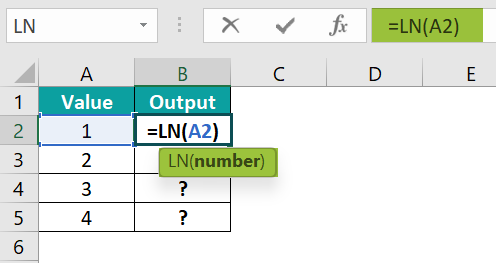5. Then, press Enter key.

Clearly, we can see that the function has returned the result in cell B2 as 0.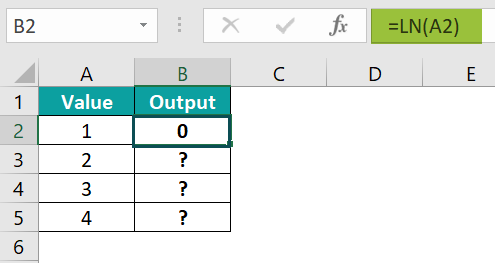6. Now, drag the cursor to cell B5, as shown in the following image.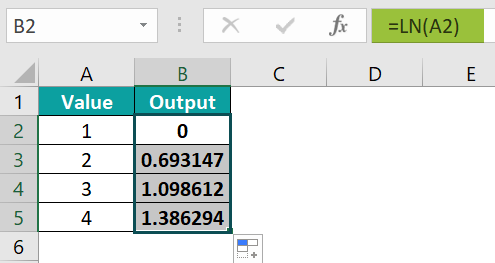Similarly, we can use LN Excel Function to get the log value.

### Examples

#### Example #1

The following table shows the fractional values in column A. We need to use the below steps to find log value using LN Excel Function.

The steps to evaluate the values by LN Function are as follows:

• Step 1: To begin with, select the cell where we will enter the formula and calculate the result. The selected cell, in this case, is cell B2.
• Step 2: Next, we will enter the LN formula in cell B2.
• Step 3: Enter the value of ‘number’ as A2, i.e., the fraction number value.
• Step 4: So, the complete formula is =LN(A2) in cell B2.
• Step 5: Press the Enter key.

Clearly, we can see that the function has returned the result in cell B2 as 0.207.

• Step 6: Next, drag the cursor to cell B5, as shown in the following image.

Similarly, we can use the function to find the log values.

#### Example #2

The below table shows zero value in cell A2. We need to use the below steps to calculate the log values with LN Excel Function.

The steps to evaluate the values by the LN Function are as follows:

• Step 1: First, select the cell where we will enter the formula and calculate the result. The selected cell, in this case, is cell B2.
• Step 2: Next, we will enter the LN formula in cell B2.
• Step 3: Then, enter the value of ‘number’ as A2, i.e., the zero value.
• Step 4: So, the complete formula is =LN(A2) in cell B2.
• Step 5: Finally, press Enter key.

Clearly, we can see that the function has returned the result in cell B2 as #NUM! error.

Likewise, we can use the function to obtain log values.

#### Example #3

The following table shows non-numeric value in cell A2. We need to use the below steps to calculate the log values with LN Excel Function.

The steps to evaluate the values by the LN Function are as follows:

• Step 1: First, select the cell where we will enter the formula and calculate the result. So, the selected cell, in this case, is cell B2.
• Step 2: Next, we will enter the LN formula in cell B2.
• Step 3: Then, enter the value of ‘number’ as A2, i.e., the non-numeric value.
• Step 4: So, the complete formula is =LN(A2) in cell B2.
• Step 5: Next, press Enter key.

So, we can see that the function has returned the result in cell B2 as #VALUE! error.

Likewise, we can use the function.

#### Example #4

The below table shows negative number value in cell A2. We need to use the below steps to calculate the log values with LN Excel Function.

The steps to evaluate the values by the LN Function in Excel are as follows:

• Step 1: First, select the cell where we will enter the formula and calculate the result. The selected cell, in this case, is cell B2.
• Step 2: Next, we will enter the LN formula in cell B2.
• Step 3: Then, enter the value of ‘number’ as A2, i.e., the negative number value.
• Step 4: So, the complete formula is =LN(A2) in cell B2.
• Step 5: Press Enter key.

Clearly, we can see that the function has returned the result in cell B2 as #NUM! error.

Similarly, we can use LN Function to find the log values.

### Important Things To Note

• The LN Excel Function is a built-in function used to calculate log values in excel.
• The function is available under Math & Trig function and accepts only a positive real number as its parameter.
• The #NUM! error occurs when the value entered as a parameter is zero.
• The #VALUE! error occurs when the parameter is a non-numeric value.
• The divisor of the number cannot be zero.
• The #NUM! error occurs when the value entered as a parameter is a negative number.

1. What does the LN function do in Excel?

The LN Function in Excel is a logarithm function in arithmetic operations. The LN Function takes a single number as the argument and shows the result as the logarithm. It is a built-in function in MS Excel. The LN Excel function is categorized under Math & Trig functions in Excel.

The syntax of the LN function is =LN(number)

2. How to calculate the LN Function in Excel?

We can insert LN Excel Function using the below steps:
Select an empty cell for the output.
Type =LN() in the selected cell. Alternatively, type =L and double-click the LN function from the list of suggestions shown by Excel.
Press Enter key.

For example, consider the below table with a single value in cell A2. We need to use the below steps to obtain the result using LN Function.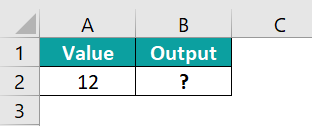The steps to evaluate the values by the LN Excel Function are as follows:

Step 1: First, select the cell where we will enter the formula and calculate the result. The selected cell, in this case, is cell B2.
Step 2: Next, we will enter the LN formula in cell B2.
Step 3: Then, enter the value of ‘number’ as A2, i.e., the single integer number value.
Step 4: So, the complete formula is =LN(A2) in cell B2.
Step 5: Next, press Enter key.
Clearly, the function has returned the result in cell B2 as 2.4849.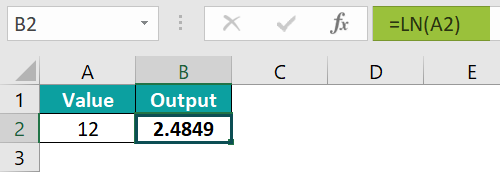Similarly, we can use LN Excel Function to fetch the log values.

3. Where is the LN Function in Excel?

We can insert LN Function in Excel using the following steps:
To begin with, choose the empty cell which will contain the result.
Next, go to the Formulas tab.
Then, select the Math & Trig option from the menu.
Now, select LN from the drop-down menu.
A window called Function Arguments appears.
As the number of arguments, enter the value in the number.
Finally, select OK.